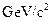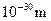Chapter 33, Problem 2PE

Chapter
Section
Textbook Problem

Calculate the mass inof a virtual carrier particle that has a range limited toby the Heisenberg uncertainty principle. Such a particle might be involved in the unification of the strong and electroweak forces.

To determine

The mass of particle in GeV/c2 of virtual carrier particle that has a range limited to 1030m by Heisenberg uncertainty principle

Explanation

Given info:

Such a particle might be involved in the unification of the strong and electroweak forces.

Formula used:

Using the expression,

ΔEΔth4π

Here, Δt is uncertainty time, ΔE is uncertainty energy and h is Plank`s constant.

Expression can also wright,

ΔE=h4πΔt=h4π(d/c)

Calculation:

We have,

h=4.14×1024GeV.s, d=1×1030and c = 3×108m/s2

Substituting the given values, we get

ΔE=(4

Still sussing out bartleby?

Check out a sample textbook solution.

See a sample solution

The Solution to Your Study Problems

Bartleby provides explanations to thousands of textbook problems written by our experts, many with advanced degrees!

Get Started

Find more solutions based on key concepts